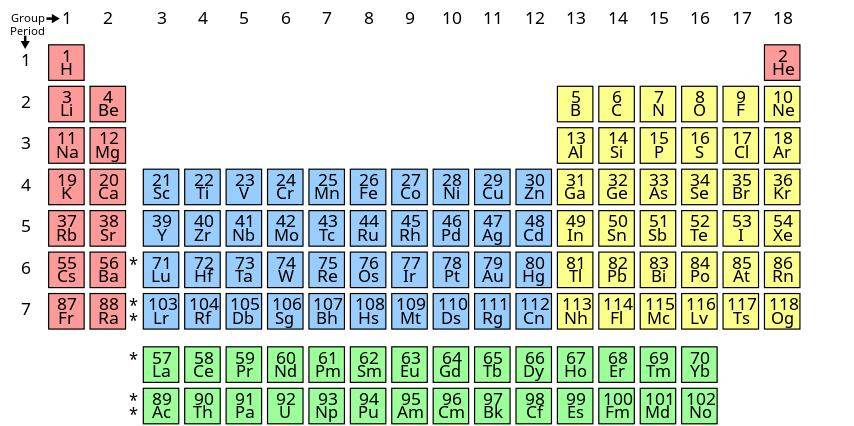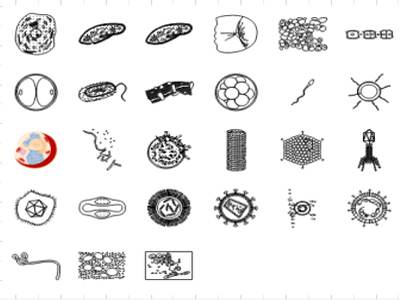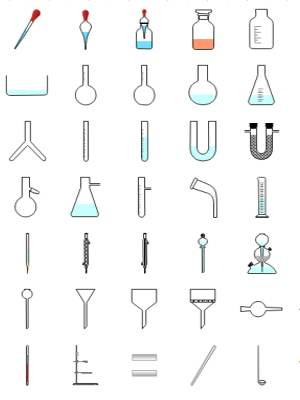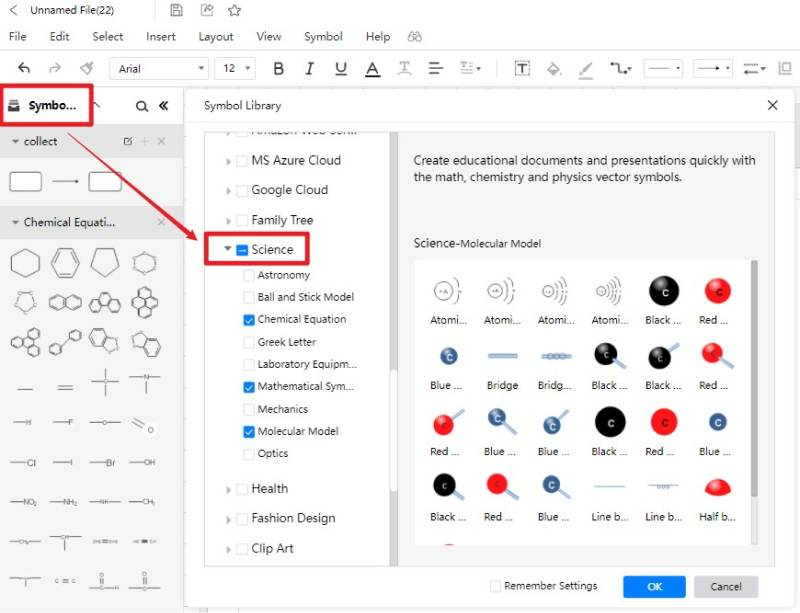# Science Symbols and Meanings

Science studies and research revolves around diagrams and experiments. Scientists use diagrams and process maps to make a detailed and accurate presentation of their observations. Using science symbols in their diagrams helps provide precise descriptions to the reader. Learn more about the benefits and uses of science diagram symbols in this article.

## 1. What Are Science Symbols

We use many symbols, signs, and units to represent various components, quantities, or calculations in science. If you are new at using and identifying science symbols, then it might seem complex to you. Science uses various symbols from other subjects like math as well. You might think that using math symbols in science makes it easier, but those symbols function differently in science.

Science represents many quantities by using initial letters from its full name that makes it easier to understand. The most common types of science symbols are either mathematic or alphabetic symbols. Having a deeper understanding of symbols helps out a lot in studies and presentations related to science.

An excellent example of why using symbols for visual representation is better than writing full names and text is that it keeps things clear and gives you more details in science diagrams. It works the same way we use emoticons on our phones to deliver messages.

## 2. Science Symbols Explained

There are various types of scientific symbols because the word science represents many disciplines and branches. Putting all of these symbols in the same category makes it hard to learn them; we divide them into some groups. You are going to learn about the most used types of symbols and signs from here.

### 2.1 Greek Alphabet Letters & Symbols

There are lots of Greek letters and symbols in modern-day science. Those who study science and its various branches mostly know about the Greek language. The modern-day English alphabet comes from a combination of Greek and Latin characters. Greek letters represent one or more quantities in science and mathematics, and using them is easy because they are similar to English alphabets.

Greek scientific symbols are mainly specified and used in various situations. Quantum mechanics, mathematics, physics use lots of Greek letters as symbols. There are also many symbols used in quantum chemistry. We also use these symbols to represent types of radiation and large quantities.

Some symbols represent life and death that are also used as Greek scientific symbols. The sign of 'Tau' depicted life in Greek history, while the sign delta represented death. Some Greek letters are not specified to a certain quantity. Following are the commonly used Greek alphabets in science.

 Upper Case Letter Lower Case Letter Greek Letter Name English Equivalent A α Alpha a B β Beta b E ε Epsilon e Z ζ Zeta z H η Eta h I ι Iota i K κ Kappa k M μ Mu m N ν Nu n O o Omicron o P ρ Rho r T τ Tau t Y υ Upsilon u X χ Chi ch Γ γ Gamma g Δ δ Delta d Θ θ Theta th Λ λ Lambda l Ξ ξ Xi x Π π Pi p Σ σ, ς * Sigma s Φ φ Phi ph Ψ ψ Psi ps Ω ω Omega o

### 2.2 Math Symbols

Mathematic symbols give you a perfect example of why it is better to use science symbols. When performing various operations or referring to mathematical quantities, math symbols make everything easy to understand. It is simple to identify and use symbols instead of the tabular or alphabetic form to explain operations.

There are different types of math symbols. We use some signs to indicate an operation, to represent specified values, and some for variables. Math symbols also depict the relationship between two quantities. We base everything in mathematics on symbols and numbers.

There are basic symbols that we use in mathematical calculations. Calculus symbols represent analytical and calculus data. We use logic symbols to represent relations and logical operations. Mathematics also uses Greek letters to name variables and some quantities. These are some of the commonly used math symbols.

 Symbol Symbol Name Meaning/definition Example = Equality sign equality 10 = 2+8 + Plus sign addition 10+20=30 - Minus sign subtraction 20-10=10 × times multiplication 3×4=12 ÷ obelus division 12 ÷6=2 ≠ Not equal sign Inequality 3 ≠ 7 ≈ Approximately equal approximation a ≈ b ± Plus-minus Both plus and minus 2 ± 9 = -7 or 11 < Strict inequality Less than 8<9 > Strict inequality Greater than 5>2 ≥ inequality Greater than or equal 5 ≥ 3x ≤ inequality Less than or equal 2x ≤ 5 ! Exclamation mark Not negation ! y π Pi Ration btw circumference and radius of a circle =3.14159 ∥ Parallel lines parallel AB ∥ CD ≅ Concurrent to Equality of geometric shapes ∆EFG≅ ∆XYZ ∑ summation Sum of values in the range / ~ Approximately equal Weak equality 8 ~ 9 ∞ Lemniscate infinity / √a Square root Taking square root √4 = ±2 ∫ integral Opposite of derivation ∫ f(y)dy ∴ therefore explain / ∵ because reason /

### 2.3 Chemical Symbols

Chemical symbols are the one or two letter notations for elements in the periodic table. In chemistry, chemical symbols are the short-hand names that represent molecules, elements, and chemical equations. The periodic table is a table of orderly arranged elements based on their atomic number. Every element is a member of one of the 18 groups in the table.

Using chemical symbols is easy as long as we know how to divide elements belonging to the same group. Elements with the same number of valance shell electrons fall under the same group in the periodic table. We also divide all elements into two significant groups metals and non-metals.Source:EdrawMax Template Community

### 2.4 Physics Symbols

Physics symbols are the notations that we use in physics to represent quantities. Many physics symbols are easy to understand because they are the initial letters from the name of those quantities. We also use SI units with quantities to avoid confusion. There are two types of physical quantities, scalar, and vector.

Scalers are those quantities that only have a magnitude with no direction. An example of scalers is time. We use seconds to represent time, and it doesn't move in some particular direction. Vector are those quantities that have both the magnitude and direction. These are some of the fundamental physical quantities with their symbols and SL units.

 Physical quantity Symbols Unit Scaler or Vector Mass m Kg Scaler Distance d, r M Scaler Volume V m3 Scaler Area A m2 Scaler Time t s Scaler Density D kg/m3 Scaler Heat flux Q J Vector Frequency f Hz Scaler Temperature T K Scaler Wavelength λ m Scaler Speed of light c m/s Scaler Angular Displacement θ rad Scaler

### 2.5 Biology Symbols

There are various fields of study in biology. We refer to biology as a study of life. We use biology symbols to represent countless living organisms, their physiologies, morphologies, and origin in this branch of science. Biological studies depend on diagrams and internal structures of organisms, and these diagrams use biological signs to depict observations.

In biology, you can’t explain structures without making a diagram with labels and detailed information. We also use initial alphabets from names as notations. There are various types of cell structures in biology. Using cell symbols makes it easier to identify the organisms without using their full scientific names.Source: EdrawMax Online

### 2.6 Lab Symbols

Lab symbols depict information about lab experiments. They tell us the type of lab instruments used in the experiments, and we also use them to promote safe handling or give warnings for anything hazardous. Safety symbols are essential when it comes to dangerous chemicals and unknown experiments.

Special apparatus is essential when it comes to laboratories. Without the right equipment, you can't perform any experiment or testing. These are some of the typical lab equipment that you can find in any working laboratory. A microscope lets you observe things that you can't see with the naked eye. You can’t keep safe samples if you don’t have test tubes. Watch glass is the best container to keep solids or to evaporate liquid.Source: EdrawMax Online

## 3. Tips for Using Science Symbols

If you want to make a scientific presentation, then using process maps is the best option. You can use science symbols to label and represent every element in the process map. Getting symbols for a process map is simple. All you have to do is visit EdrawMax Online’s library and create a detailed presentation using their countless scientific symbols. Follow these steps to get to science diagrams in EdrawMax quickly.

### 3.1 Use a Professional Science Diagram Maker

You can find science symbols from EdrawMax Online>Symbol Library> Science.### 3.2 Create Symbols by Yourself

If you don't find one of the symbols you are looking for, you can easily import it or draw your symbol using EdrawMax Online. You can refer this video to learn more.YouTube.

## 4. FAQ about Science symbols

1. How to Type Math Symbols?

You can write math equations and symbols with EdrawMax’s math editor. You can also import symbols from the web or draw them yourself if you don't find them in the library.

2. How to create a science diagram?

You can easily create a science diagram by using EdrawMax’s process map and science symbols. All you have to do is visit EdrawMax Online and start making your science diagram.

## 5. Conclusion

Everything related to science depends on scientific symbols. You can easily make a science diagram by using science symbols. You can use physics symbols, math symbols, and Greek symbols to represent quantities and chemicals. If you want to make a detailed biological diagram, then using biology symbols makes it easier to explain to the reader. EdrawMax Online is an excellent tool because of its wide support and extensive features like comprehensive symbols library, science graphic organizer examples, export in multiple formats, and import of symbols and libraries. It is available for free online and desktop with maximum feature support.

## Create and share 280+ diagrams with EdrawMax Online

Related Articles